# XLOOKUP

If you have Excel 365 or Excel 2021, use XLOOKUP instead of VLOOKUP. The XLOOKUP function is easier to use and has some additional advantages.

### Exact Match

By default, the XLOOKUP function in Excel 365/2021 performs an exact match.

1. The XLOOKUP function below looks up the value 53 (first argument) in the range B3:B9 (second argument).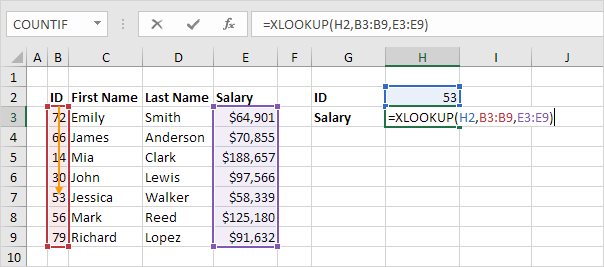2. Next, it simply returns the value in the same row from the range E3:E9 (third argument).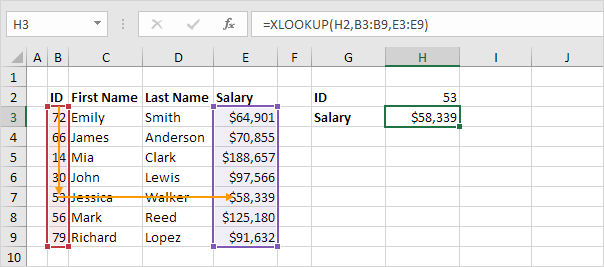3. Here's another example. Instead of returning the salary, the XLOOKUP function below returns the last name (replace E3:E9 with D3:D9) of ID 79.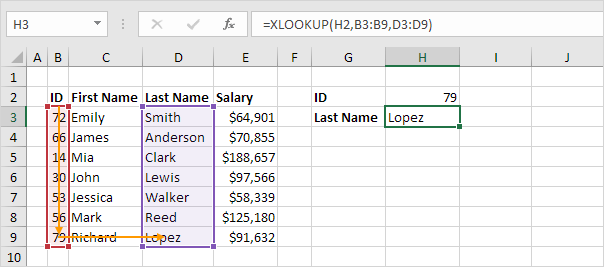If the XLOOKUP function cannot find a match, it returns a #N/A error.

1. For example, the XLOOKUP function below cannot find the value 28 in the range B3:B9.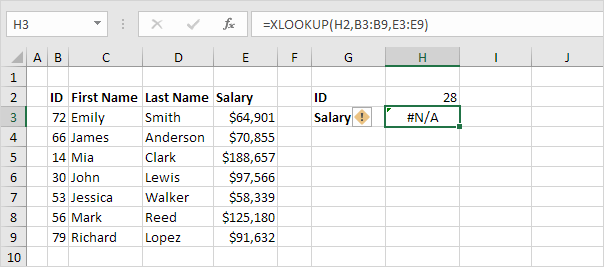2. Use the fourth argument of the XLOOKUP function to replace the #N/A error with a friendly message.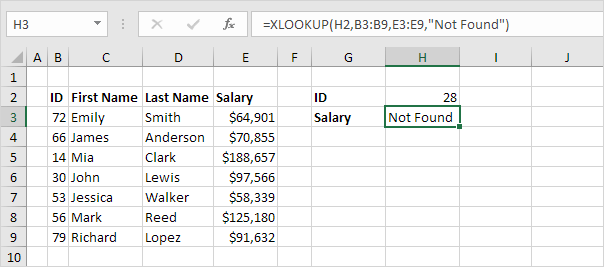### Approximate Match

Let's take a look at an example of the XLOOKUP function in approximate match mode.

1. The XLOOKUP function below looks up the value 85 (first argument) in the range B3:B7 (second argument). There's just one problem. There's no value 85 in this range.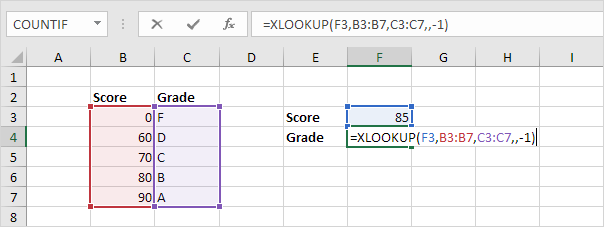2. Fortunately, the value -1 (fifth argument) tells the XLOOKUP function to find the next smaller value. In this example, the value 80.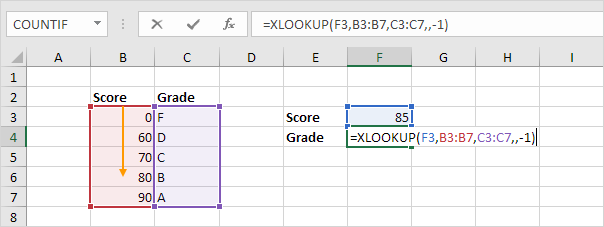3. Next, it simply returns the value in the same row from the range C3:C7 (third argument).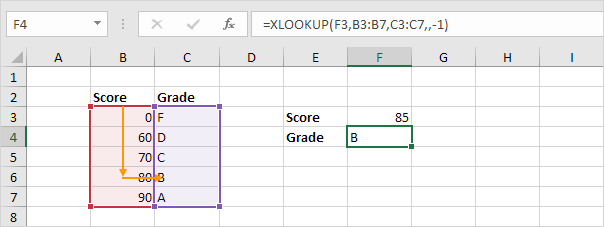Note: use 1 instead of -1 for the fifth argument to find the next larger value. In this example, the value 90. The XLOOKUP function also works with unsorted data. In this example, there's no need to sort the scores in ascending order.

### Left Lookup

Instead of using INDEX and MATCH in Excel to perform a left lookup, simply use the XLOOKUP function. For example, take a look at the XLOOKUP function below.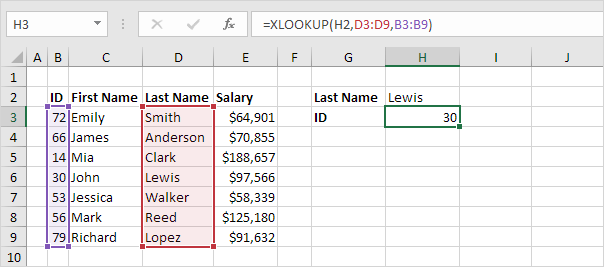Explanation: the XLOOKUP function looks up the last name and returns the ID.

### Multiple Values

The XLOOKUP function in Excel 365/2021 can return multiple values.

1. First, the XLOOKUP function below looks up the ID and returns the first name (nothing new).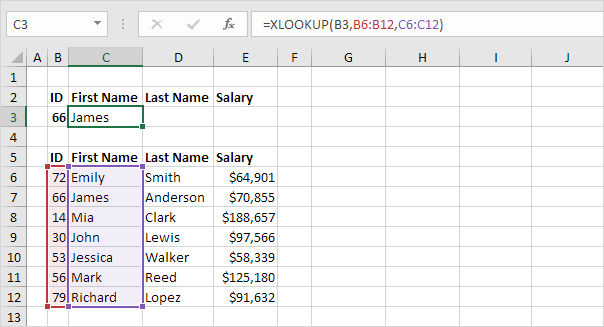2. Replace C6:C12 with C6:E12 to return the first name, last name and salary.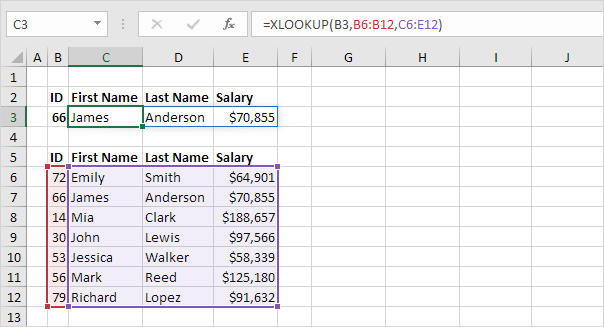Note: the XLOOKUP function, entered into cell C3, fills multiple cells. Wow! This behavior in Excel 365/2021 is called spilling.

### Horizontal Lookup

Instead of using the HLOOKUP function in Excel to perform a horizontal lookup, simply use the XLOOKUP function.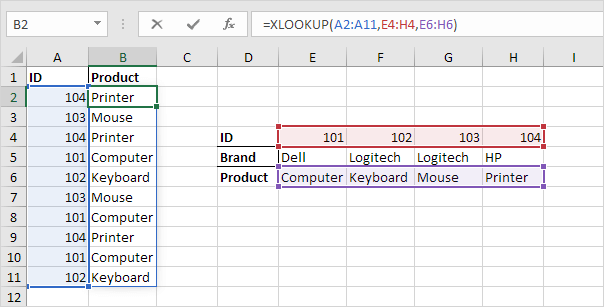Note: the XLOOKUP function, entered into cell B2, fills multiple cells. Wow! This behavior in Excel 365/2021 is called spilling.

### Last Match

By default, the XLOOKUP function performs a first to last search (nothing new). To perform a last to first search, set the sixth argument to -1 (see example below).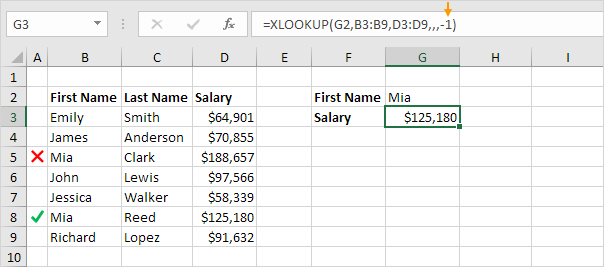Explanation: this XLOOKUP function returns the salary of Mia Reed, not Mia Clark.

Go to Next Chapter: Financial Functions